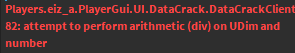# How can I detect if a frame is touching another frame?

Hello,

So, in the image I provided below, it shows 8 frames with a red bar in the middle. If a frame is clicked and is on the red line, it’ll go to the next. But my question is, if a frame isn’t on the red line, how could I make the gui disappear?

If you know something that can help, please comment on what I can do to accomplish this, thank you, if not have a great rest of your day!

I don’t generally work with UI, but I suggest you could use (and this is in pseudocode):

if frame.yPos - (frame.Height / 2) < redline.yPos + (redline.Height / 2) and frame.yPos + (frame.Height / 2) > redline.yPos - (redline.Height / 2) then
fireEvent()

theres probably something wrong with my math there because its half past two in the morning but im sure you understand what im getting at.1 Like

How could I get multiple frames? Would a for loop work? Or is there another way I’d have to do it?

EDIT: Also it’s saying that yPos is not a valid member of frame.

the actual y position property of a frame is frame.Position.Y i believe

What is Height? That is also giving me an error as well, sorry for all the questions I’m asking, I just genuinely have no idea how to do math like this lol.

It’s fine. I used height to refer to the size of the “redLine” frame on the y axis.

So, would I do `redLine.Position.Y`?

`redLine.Size.Y` if that is what you have named the red line

Ohh okay, ill test this out now and let you know. Thank you!

I’m getting this error:Here’s my code:

``````Lines.InputBegan:Connect(function(input, gameProcessedEvent)
for i,v in pairs(Lines:GetChildren()) do
if v.Name ~= "MID" then
local redline = Lines.MID
if input.UserInputType == Enum.UserInputType.MouseButton1 and v.Position.Y - (v.Size.Y / 2) < redline.Position.Y + (redline.Size.Y / 2) and v.Position.Y + (v.Size.Y / 2) > redline.Position.Y - (redline.Size.Y / 2) then
if LineValues.First.Value == false then
LineValues.First.Value = true
tweens.First:Pause()
elseif LineValues.Second.Value == false then
LineValues.Second.Value = true
tweens.Second:Pause()
elseif LineValues.Third.Value == false then
LineValues.Third.Value = true
tweens.Third:Pause()
elseif LineValues.Fourth.Value == false then
LineValues.Fourth.Value = true
tweens.Fourth:Pause()
elseif LineValues.Fifth.Value == false then
LineValues.Fifth.Value = true
tweens.Fifth:Pause()
elseif LineValues.Sixth.Value == false then
LineValues.Sixth.Value = true
tweens.Sixth:Pause()
elseif LineValues.Seventh.Value == false then
LineValues.Seventh.Value = true
tweens.Seventh:Pause()
elseif LineValues.Eighth.Value == false then
LineValues.Eighth.Value = true
tweens.Eighth:Pause()
-- tweens["TweenName"]:Cancel() returns all frames to starting position
else
--	Failed()
end
end
end
end
end)
````````

i apologise i probably cant help you any further. the math i believe is correct however the error shows that its incorrectly implemented into the code and I’m only just relearning LuaU so I’ve got no idea how to fix that. sorry! good luckOkay, thank you for getting me this farI’ll ask around and see if I can figure it out.

1 Like